libxlsxwriter
chart_data_tools.c

A demo of an various Excel chart data tools that are available via a libxlsxwriter chart. These include Drop Lines and High-Low Lines.

Chart 1: chart with high-low lines.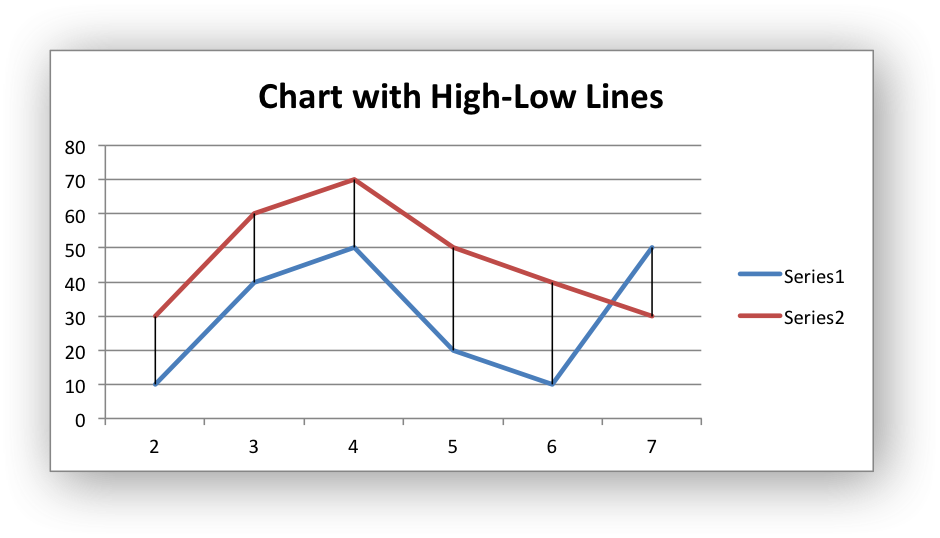Chart 2: chart with drop lines.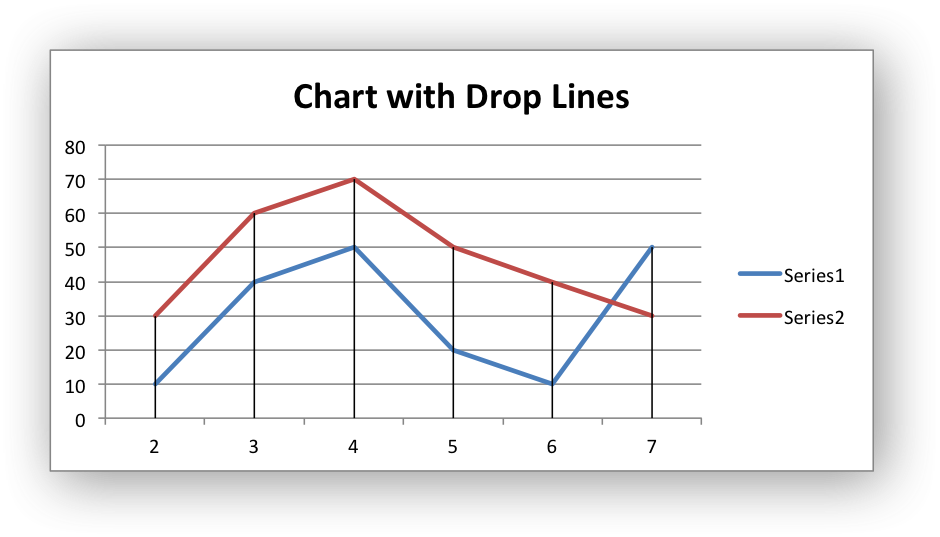Chart 3: chart with up-down bars.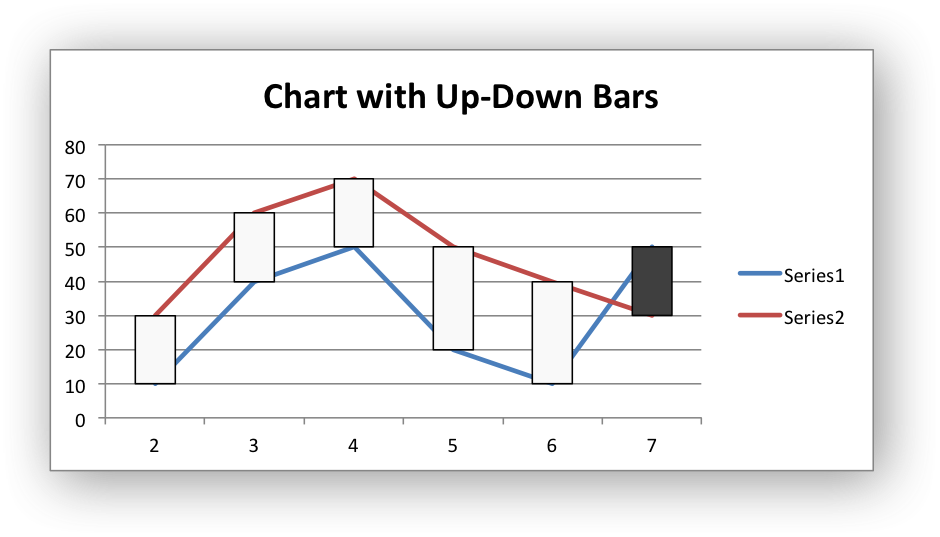Chart 4: chart with formatted Up-down bars.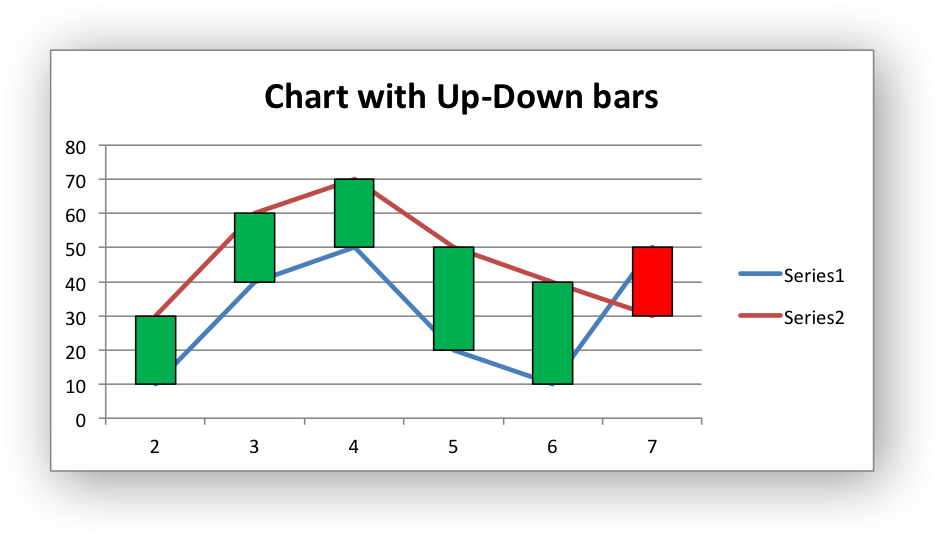Chart 5: chart with markers and data labels.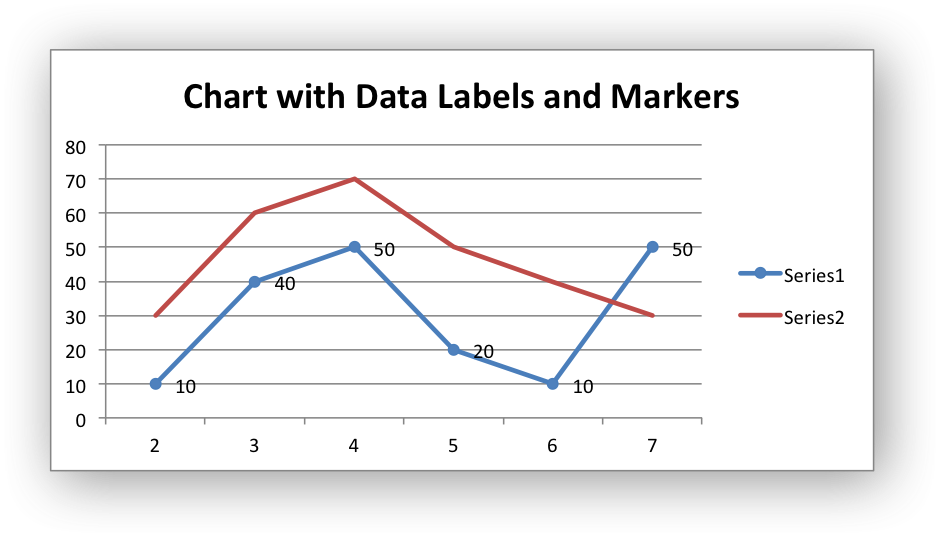Chart 6: chart with error bars.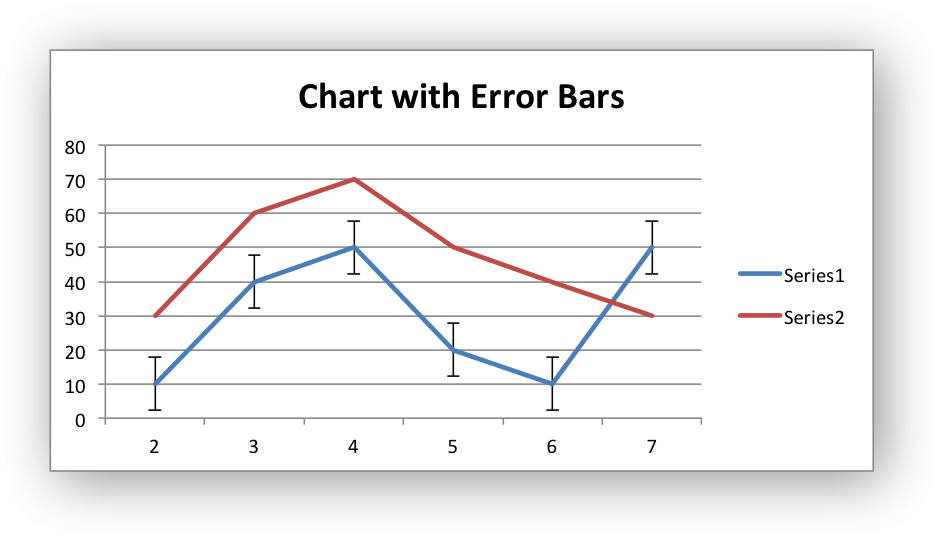Chart 7: chart with a trendline.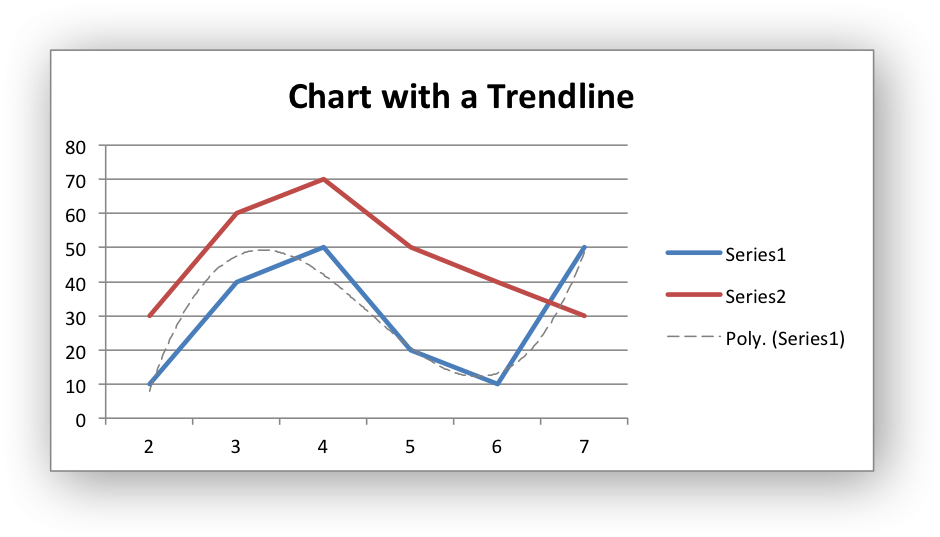/*
* A demo of an various Excel chart data tools that are available via a
* libxlsxwriter chart.
*
* These include Drop Lines and High-Low Lines.
*
* Copyright 2014-2021, John McNamara, jmcnamara@cpan.org
*
*/
#include "xlsxwriter.h"
/*
* Write some data to the worksheet.
*/
void write_worksheet_data(lxw_worksheet *worksheet, lxw_format *bold) {
int row, col;
uint8_t data = {
/* Three columns of data. */
{2, 10, 30},
{3, 40, 60},
{4, 50, 70},
{5, 20, 50},
{6, 10, 40},
{7, 50, 30}
};
worksheet_write_string(worksheet, CELL("A1"), "Number", bold);
worksheet_write_string(worksheet, CELL("B1"), "Batch 1", bold);
worksheet_write_string(worksheet, CELL("C1"), "Batch 2", bold);
for (row = 0; row < 6; row++)
for (col = 0; col < 3; col++)
worksheet_write_number(worksheet, row + 1, col, data[row][col] , NULL);
}
/*
* Create a worksheet with examples charts.
*/
int main() {
lxw_workbook *workbook = workbook_new("chart_data_tools.xlsx");
/* Add a bold format to use to highlight the header cells. */
/* Write some data for the chart. */
write_worksheet_data(worksheet, bold);
/*
* Chart 1. Example with High Low Lines.
*/
/* Add a chart title. */
chart_title_set_name(chart, "Chart with High-Low Lines");
/* Add the first series to the chart. */
/* Add high-low lines to the chart. */
/* Insert the chart into the worksheet. */
worksheet_insert_chart(worksheet, CELL("E2"), chart);
/*
* Chart 2. Example with Drop Lines.
*/
/* Add a chart title. */
chart_title_set_name(chart, "Chart with Drop Lines");
/* Add the first series to the chart. */
/* Add drop lines to the chart. */
chart_set_drop_lines(chart, NULL);
/* Insert the chart into the worksheet. */
worksheet_insert_chart(worksheet, CELL("E18"), chart);
/*
* Chart 3. Example with Up-Down bars.
*/
/* Add a chart title. */
chart_title_set_name(chart, "Chart with Up-Down bars");
/* Add the first series to the chart. */
/* Add Up-Down bars to the chart. */
/* Insert the chart into the worksheet. */
worksheet_insert_chart(worksheet, CELL("E34"), chart);
/*
* Chart 4. Example with Up-Down bars with formatting.
*/
/* Add a chart title. */
chart_title_set_name(chart, "Chart with Up-Down bars");
/* Add the first series to the chart. */
/* Add Up-Down bars to the chart, with formatting. */
lxw_chart_fill up_fill = {.color = 0x00B050};
chart_set_up_down_bars_format(chart, &line, &up_fill, &line, &down_fill);
/* Insert the chart into the worksheet. */
worksheet_insert_chart(worksheet, CELL("E50"), chart);
/*
* Chart 5. Example with Markers and data labels.
*/
/* Add a chart title. */
chart_title_set_name(chart, "Chart with Data Labels and Markers");
/* Add the first series to the chart. */
/* Add series data labels. */
/* Insert the chart into the worksheet. */
worksheet_insert_chart(worksheet, CELL("E66"), chart);
/*
* Chart 6. Example with Error Bars.
*/
/* Add a chart title. */
chart_title_set_name(chart, "Chart with Error Bars");
/* Add the first series to the chart. */
/* Add error bars to show Standard Error. */
chart_series_set_error_bars(series->y_error_bars,
/* Add series data labels. */
/* Insert the chart into the worksheet. */
worksheet_insert_chart(worksheet, CELL("E82"), chart);
/*
* Chart 7. Example with a trendline
*/
/* Add a chart title. */
chart_title_set_name(chart, "Chart with a Trendline");
/* Add the first series to the chart. */
/* Add a polynomial trendline. */
chart_series_set_trendline_line(series, &poly_line);
/* Insert the chart into the worksheet. */
worksheet_insert_chart(worksheet, CELL("E98"), chart);
return workbook_close(workbook);
}
chart_series_set_marker_type
void chart_series_set_marker_type(lxw_chart_series *series, uint8_t type)
Set the data marker type for a series.
LXW_CHART_LINE_DASH_LONG_DASH
@ LXW_CHART_LINE_DASH_LONG_DASH
Definition: chart.h:218
workbook_close
lxw_error workbook_close(lxw_workbook *workbook)
Close the Workbook object and write the XLSX file.
chart_series_set_trendline
void chart_series_set_trendline(lxw_chart_series *series, uint8_t type, uint8_t value)
Turn on a trendline for a chart data series.
chart_series_set_trendline_line
void chart_series_set_trendline_line(lxw_chart_series *series, lxw_chart_line *line)
Set the trendline line properties for a chart data series.
LXW_CHART_MARKER_CIRCLE
@ LXW_CHART_MARKER_CIRCLE
Definition: chart.h:265
lxw_chart_series * chart_add_series(lxw_chart *chart, const char *categories, const char *values)
Add a data series to a chart.
workbook_new
lxw_workbook * workbook_new(const char *filename)
Create a new workbook object.
LXW_CHART_ERROR_BAR_TYPE_STD_ERROR
@ LXW_CHART_ERROR_BAR_TYPE_STD_ERROR
Definition: chart.h:868
chart_series_set_error_bars
void chart_series_set_error_bars(lxw_series_error_bars *error_bars, uint8_t type, double value)
format_set_bold
void format_set_bold(lxw_format *format)
Turn on bold for the format font.
lxw_chart_line
Struct to represent a chart line.
Definition: chart.h:639
chart_set_high_low_lines
void chart_set_high_low_lines(lxw_chart *chart, lxw_chart_line *line)
Turn on and format high-low Lines for a chart.
chart_set_up_down_bars
void chart_set_up_down_bars(lxw_chart *chart)
Turn on up-down bars for the chart.
LXW_CHART_LINE
@ LXW_CHART_LINE
Definition: chart.h:125
lxw_worksheet
Struct to represent an Excel worksheet.
Definition: worksheet.h:2107
LXW_COLOR_BLACK
@ LXW_COLOR_BLACK
Definition: format.h:181
LXW_CHART_TRENDLINE_TYPE_POLY
@ LXW_CHART_TRENDLINE_TYPE_POLY
Definition: chart.h:941
lxw_format
Struct to represent the formatting properties of an Excel format.
Definition: format.h:358
lxw_chart
Struct to represent an Excel chart.
Definition: chart.h:1090
worksheet_insert_chart
lxw_error worksheet_insert_chart(lxw_worksheet *worksheet, lxw_row_t row, lxw_col_t col, lxw_chart *chart)
Insert a chart object into a worksheet.
lxw_chart_series
Struct to represent an Excel chart data series.
Definition: chart.h:960
lxw_chart_fill::color
lxw_color_t color
Definition: chart.h:666
LXW_COLOR_GRAY
@ LXW_COLOR_GRAY
Definition: format.h:193
chart_set_up_down_bars_format
void chart_set_up_down_bars_format(lxw_chart *chart, lxw_chart_line *up_bar_line, lxw_chart_fill *up_bar_fill, lxw_chart_line *down_bar_line, lxw_chart_fill *down_bar_fill)
Turn on up-down bars for the chart, with formatting.
chart_series_set_labels
void chart_series_set_labels(lxw_chart_series *series)
Add data labels to a chart series.
lxw_chart_fill
Struct to represent a chart fill.
Definition: chart.h:663
lxw_chart_line::color
lxw_color_t color
Definition: chart.h:642
lxw_workbook
Struct to represent an Excel workbook.
Definition: workbook.h:292
chart_title_set_name
void chart_title_set_name(lxw_chart *chart, const char *name)
Set the title of the chart.
worksheet_write_string
lxw_error worksheet_write_string(lxw_worksheet *worksheet, lxw_row_t row, lxw_col_t col, const char *string, lxw_format *format)
Write a string to a worksheet cell.
LXW_COLOR_RED
@ LXW_COLOR_RED
Definition: format.h:217
worksheet_write_number
lxw_error worksheet_write_number(lxw_worksheet *worksheet, lxw_row_t row, lxw_col_t col, double number, lxw_format *format)
Write a number to a worksheet cell.
CELL
#define CELL(cell)
Convert an Excel A1 cell string into a (row, col) pair.
Definition: utility.h:45